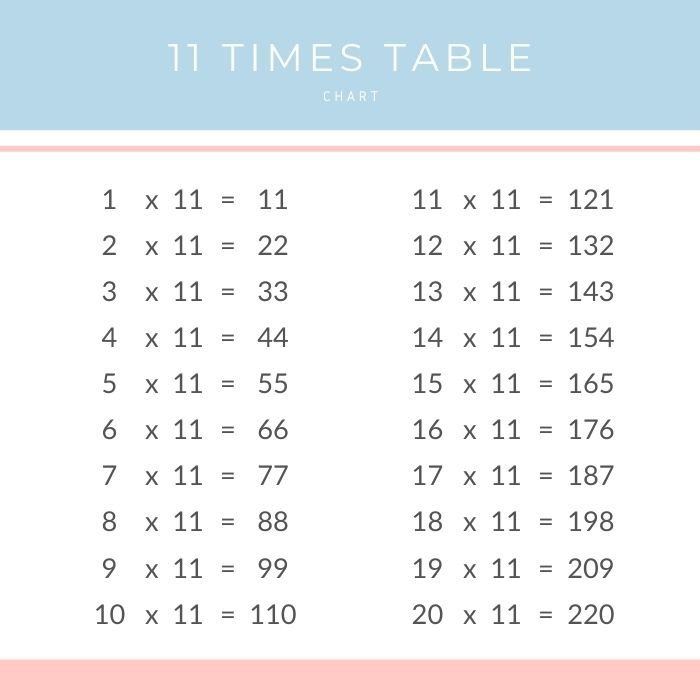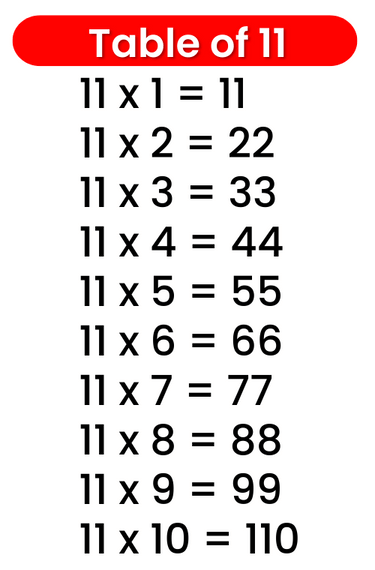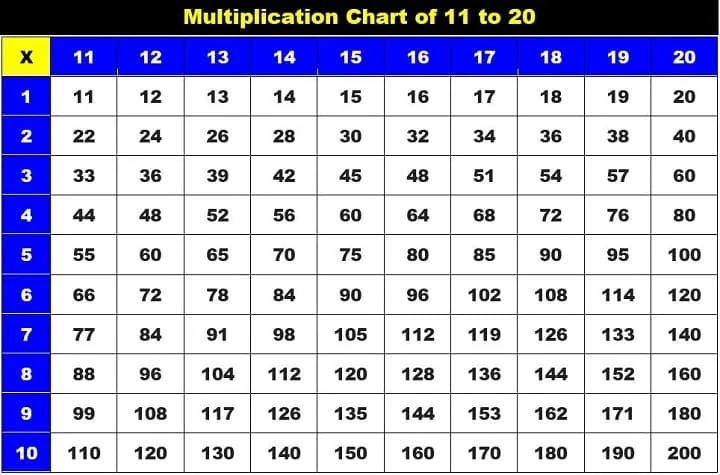# Times Table of 11, 11 ka table

## Times Table of 11, 11 ka table

Maths Multiplication Table of 11, Times Table of 11, 11 ka table, 11 ki Table Chart

11 ×‌‌ 1 = 11
11 ×‌‌ 2 = 22
11 ×‌‌ 3 = 33
11 ×‌ 4 = 44
11 ×‌ 5 = 55
11 ×‌ 6 = 66
11 ×‌ 7 = 77
11 ×‌ 8 = 88
11 ×‌ 9 = 99
11 ×‌ 10 = 110

## 11 ka table### Multiplication Tables of eleven upto 20### Times Table of 11 chart